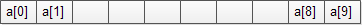# C Array

Summary: in this tutorial, you will learn about the C array, how to declare arrays and how to manipulate array’s elements effectively.

## C Array Definition

C array is a variable that holds multiple elements which share the same data type.

## Declaring C Arrays

In order to declare an array, you need to specify:

• The data type of the array’s elements. It could be int, float, char, etc.
• The name of the array.
• A fixed number of elements that array may contain. The number of elements is placed inside square brackets followed the array name.

The following illustrates the typical syntax of declaring an array:

For example, you can declare an array that holds 10 integers as follows:

When you declare an array, the compiler allocates a memory block that holds the entire array. The array elements are stored sequentially in the memory as the following picture:You must follow the following rules when you declare an array in C:

## Accessing array elements

You can access an individual element of the array by using the array name followed by the element subscript enclosed in square brackets as follows:

Notice that the array elements start from `0`, not `1`, so the first element of the `a` array is `a` and the last element is `a[size-1] `where `size `is the number of element in the `a` array.

The following program demonstrates how to access elements of an array:

## Initializing arrays

You can initialize an array like a variable. To initialize an array, you provide default values enclosed in curly braces in the declaration part and assign it to the array. The following example initializes an array of 5 integers.

If you omit the size of the array, the compiler will create an array with the size that is sufficient enough to hold the initialized values. See the following example:

The compiler will create an array with the size of three.

If you initialize just only few array elements, you will not know exact values of the uninitialized elements when the program executes e.g.

The `a` and `a` can hold any integer values.

## Array and Pointer

The array name is equivalent to a pointer that points to the first element in the array. When you dereference the array name, you get the value of the first element.

For example, when you declare:

You are actually declaring a pointer named `a` that points to the first element in the array i.e., `a` is exactly is `&a`

The output is as we expected:

However the array name is treated as a constant pointer i.e., you cannot change the memory address where it points to. The expression a[i] is equivalent to *(a + i) . This gives you the possibility of accessing elements of the array using not only a subscript but also a pointer.

The following program helps you understand more about accessing elements of an array using a pointer.

The following is output:

## C Multidimensional Array

### Declare C multidimensional array

C multidimensional array has more than one subscript. To declare a multidimensional array, you use the following syntax:

Let’s examine the syntax above:

• First, you specify the data type for the elements of the multidimensional array. Of course, it can be any valid data type such as int, character, float, pointer, struct, etc.
• Second, you provide the name of the multidimensional array. The name should follow the naming rules of variables.
• Third, you determine the multidimensional array’s dimensions and their sizes. The number of dimensions depends on C compiler. Typically, twelve is very common. However, it would be more complex if you have more than three dimensions.

### Multidimensional array example

In the following example, first, we declare a two-dimensional array of integers with two rows and three columns. Next, we use the `scanf()` function to read the number from user’s inputs. Then, we display the array content on the screen.

### Initializing Multidimensional Arrays

The following example illustrates how to initialize a multidimensional array of integers with three columns and three rows:

### Multidimensional array and function

C provides you with two ways that allow you to pass a multidimensional array to a function:

• For two-dimensional arrays, you should always pass the number of columns to the function. Notice that function does not need to know a number of rows. For three or more dimensional array, only the first dimension can be omitted.
• You can pass a pointer to an array to a function.

The following example demonstrates two ways of passing a multidimensional array to a function.

The following is the output of the program:

In this tutorial, you have learned about the array, which is a complex type in C that allows you to store multiple elements with the same data type. Next, you learned about some common operations that you often work with the array including declaring and initializing arrays. Then you learned how to work with multidimensional arrays in C.